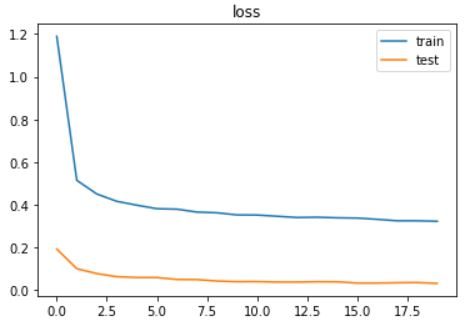# Can't explain the difference test loss vs train loss

Hi, Thanks in advance for looking into my question.
let me first describe the problem:

I have designed a very simple network to classify mnist dataset.
I have refactor as much as possible and make a clean code.
so everything ran smoothly and I would get good results on train and validation set.
until I read that If you are using dropout in your modules, then you have to use `net.train()` in training phase, and `net.eval()` when in testing.

so I added the changes in my code. now, I can’t explain test loss vs train loss.
train loss is fine, and is decreasing steadily as expected. but test loss is way much lower than train loss from the first epoch until to the end and does not change that much!
this is so weird, and I can’t find out what I am doing wrong.

for your reference I have put the loss and accuracy plots during epochs here:following, I will upload my entire code.

### imports

``````import torch
import torch.nn as nn
import torch.optim as optim
import torchvision
import torchvision.transforms as transforms
import matplotlib.pyplot as plt
``````

### Defining Network (Custom nn.Module)

``````class Flatten(nn.Module):
def forward(self, x):
batch_size = x.size()
return x.view(batch_size, -1)

class Net(nn.Module):
def __init__(self):
super(Net, self).__init__()
self.conv1 = nn.Conv2d(1, 6, 3)
self.conv2 = nn.Conv2d(6, 16, 5)
self.maxpool = nn.MaxPool2d(2, 2)
self.relu = nn.ReLU()
self.flatten = Flatten()
self.dropout = nn.Dropout(0.25)
self.fc1 = nn.Linear(256, 64)
self.fc2 = nn.Linear(64, 10)

def forward(self, x):
x = self.maxpool(self.relu(self.conv1(x)))
x = self.maxpool(self.relu(self.conv2(x)))
x = self.flatten(x)
x = self.relu(self.dropout(self.fc1(x)))
x = self.dropout(self.fc2(x))
return x
``````

### Problem Class

this class, contains the logic of training and validation. this is just for cleaner code.

``````class Problem:
def __init__(self, net, criterion, opt, device):
self.net = net
self.criterion = criterion
self.opt = opt
self.device = device

def count_correct(self, y_pred, y):

def predict(self, X):
self.net.eval()
return self.net(X)

losses = []
accs = []
test_losses = []
test_accs = []
for epoch in range(epochs):
self.net.train()

correct_predictions = 0
total_loss = 0

X, y = data.to(self.device), data.to(self.device)
y_pred = self.net(X)
loss = self.criterion(y_pred, y)
loss.backward()
self.opt.step()

correct_predictions += self.count_correct(y_pred, y).item()
total_loss += loss.item()

losses.append(total_loss)
accs.append(correct_predictions)

test_losses.append(test_loss)
test_accs.append(test_acc)

if verbose == 1 and testloader is not None:
print("Epoch: {}; Loss: {}; Acc: {}; Test Loss: {}; Test Acc: {}"
.format(epoch, total_loss, correct_predictions, test_loss, test_acc))
elif verbose == 1:
print("Epoch: {}; Loss: {}; Acc: {}".format(epoch, total_loss, correct_predictions))

return losses, accs, test_losses, test_accs

self.net.eval()
total_loss = 0
correct_predictions = 0
X, y = data.to(self.device), data.to(self.device)
y_pred = self.net(X)
loss = self.criterion(y_pred, y)

total_loss += loss.item()
correct_predictions += self.count_correct(y_pred, y).item()

``````

### Creating Instances and Training the Model

``````# configurations
lr = 0.01
batch_size = 250
epochs = 20
device = torch.device("cuda") if torch.cuda.is_available() else torch.device("cpu")

# preparing train and test dataloaders
transform = transforms.Compose([transforms.ToTensor(), transforms.Normalize((0.5,), (0.5,))])

# creating instances
net = Net()
net.to(device)
criterion = nn.CrossEntropyLoss()
opt = optim.SGD(net.parameters(), lr, 0.9)
problem = Problem(net, criterion, opt, device)

# let model train

# plotting test/train loss
plt.title('loss')
plt.plot(train_losses), plt.plot(test_losses)
plt.legend(['train', 'test'])
plt.show()

# plotting test/train accuracy
plt.title('accuracy')
plt.plot(train_accs), plt.plot(test_accs)
plt.legend(['train', 'test'])
plt.show()
``````

I don’t think there is something wrong with this. Also I think it is really good that test loss is much lower which means its generalizing well. Here is a little explanation why?
Dropout layer sets some of features to 0. And gradient does not flow backward through those features. In your case 25% of features are dropped making it harder for model to make predictions. In evaluation dropout layer does nothing, making it easier for model to predict. It very rough explanation but something like this happens. If you increase features (e.g increase number of out_channels from 16 to 32 in conv2) train accuracy will increase if thats what you want.

so you don’t suspect anything is wrong?
I understand your explanation, but aren’t the activation maps divided by 1/p to account for those dropped out neurons?
I might not understand it, but at least I want to make sure that I am not seeing this because I have done something wrong.

Try putting net.train() in place of net.eval() for testing. See if that brings both losses equal.
If dropout is the only thing that caused this change. You shouldn’t worry. Again try increasing features in each layer, it should bring both curves closer.

I have done that, and this problem goes away.
but why this problem exists when net.eval() is used when computing loss for validation set?

This is not a problem. This is normal. When there are not much feature to account for dropped one, something like this can happen.

1 Like